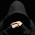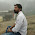# Java Program For Find The Gross Salary of an Employee

Problem:- Calculate gross salary in Java or Calculate Net Salary Calculate Gross salary Deduction or Program To Calculate And Display Employee Salary or Java Program to Calculate Salary of an Employee or Write A C Program For Find The Gross Salary Of An Employee .gross salary is different from Net, Salary gross salary calculated annual basis we can calculate a gross salary by using the following formula (Gross salary = Net Salary - Deduction. ).

Deduction = Tax ( HRA. + DA. + MA. ).

Check This:- Hacker rank solution for C++ Domain.

Formula's:-

Gross Salary = Basic + Da + Hra + Ma.

Deduction = Gross Salary - Pf - Pt - It.

Net Salary = Gross Salary - Deduction.

See Also:- C++ Program For Find The Gross Salary of an Employee

Logic:- For finding a gross salary of Employee we need to calculate DA and HRA Then Sum Of Basic Salary + HRA + DA. after calculating the sum print the sum. So basically first we calculate a DA, and HRA after that we add basic salary + HRA +DA so, in this way, we can find the Gross salary of an employee.

### Java Program For Find The Gross Salary Of An Employee

```import java.util.Scanner;
/* Program By Ghanendra Yadav
Visit http://www.programmingwithbasics.com/
*/
class employeesalary
{
public static void main(String args[])
{
float basic_salary,da,hra,da1,hra1,GrossPayment;

Scanner scan=new Scanner(System.in);

System.out.println("Enter Basic Salary Of Employee: ");
basic_salary=scan.nextFloat();

System.out.println("Enter Basic DA Of Employee: ");
da1=scan.nextFloat();

System.out.println("Enter Basic HRA Of Employee: ");
hra1=scan.nextFloat();

da = (da1 * basic_salary) / 100;
hra = (hra1 * basic_salary) / 100;

GrossPayment = basic_salary + da + hra;

System.out.println("Gross Salary Of Employee: "+GrossPayment);
}
}
```

See Also:- C Program For Find The Gross Salary Of An Employee

Output:-

1.write a code in java that input salary and grade of an employee and apply below conditions:
i) in case of grade 15 or above than bonus is 15%
ii) in case of grade 16 or above than bonus is 20%
iii) in case of grade 18 or above than bonus is 25%
iv) after calculating total salary deduct 13% GST in case that salary is 15000 or above. deduct 15% GST and in case that salary is 22000 or above.
v) Add 6% bonus at the end
Calculate net salary according to above condition and display it.

Give me solution of this question I need emergency solution of this question. Thanks

1.Thanks For Writing For Us

2.Create a program that will compute the salary deduction of an employee base on the given range.

Employee Salary Tax Deduction
15000 0
20000 0
25000 634.57
30000 1634.57
35000 2655.72

Create the following methods
1. A method that will get the user input.
Methods to be created:
-Employee name method with 1 parameter and String attribute
-Employee Salary method with 1 parameter and double attribute

2. Create a method that will deduct the tax from the employee's salary.
Methods to be created:
-Compute method with 2 parameters with an attribute of double and String
-The Compute method's body should contain a chain method. The method salary and name should be invoked inside the compute method.

3. The program should loop through a Try again question.

3.Create a program that will compute the salary deduction of an employee base on the given range.

Employee Salary Tax Deduction
15000 0
20000 0
25000 634.57
30000 1634.57
35000 2655.72

Create the following methods
1. A method that will get the user input.
Methods to be created:
-Employee name method with 1 parameter and String attribute
-Employee Salary method with 1 parameter and double attribute

2. Create a method that will deduct the tax from the employee's salary.
Methods to be created:
-Compute method with 2 parameters with an attribute of double and String
-The Compute method's body should contain a chain method. The method salary and name should be invoked inside the compute method.

3. The program should loop through a Try again question.

4.Appreciate the recommendation. Let me try it out. hotmail login

1.Thanks and Keep Visit.

5.Write an application program to create a parent class called Software with member variables totalCost and vat and a virtual method called calculateVat(). A child class called PurchasedSoftware should redefine the method in super class such that it will determine the amount of vat that will be charged on total cost of software brought in java